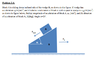# Find Magnitude & Direction for acceleration:

## Homework Statement

:
Block B has acceleration of 4 m/s2... Relative acceleration of block A w/ respect to B is 4 m/s2. Find magnitude & direction of accel for A?[/B]

## Homework Equations

a_A = a_B + a_A/B[/B]

## The Attempt at a Solution

a_A = 4 +4 = 8 m/s2 <--

8*cos(20) = 7.52 (x-component)

8*sin(20) = 2.74 (y-component)

direction= inverse tangent of (2.74 / 7.52) = 20.02 degrees <--

this was my attempt, not sure if I did it correctly. [/B]

#### Attachments

•accel2.png
12.7 KB · Views: 1,087
a_A = a_B + a_A/B
Those are vectors, you cannot add their magnitudes.